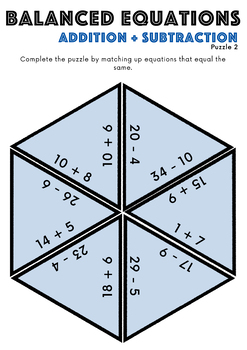# Balance The Equation Tarsia Puzzle: Addition & Subtraction - Grades 1 - 6 BundleSubject
Grade Levels
Resource Type
Product Rating
File Type

Compressed Zip File

Be sure that you have an application to open this file type before downloading and/or purchasing.

71 MB|18 pages
Share
3 Products in this Bundle
3 products
1. Use these entertaining math puzzles for your students to practice finding balanced equations with addition and subtraction. This pack contains three differentiated puzzles from simple additions to additions over 10 and 20. Each puzzle has a complete set and a muddled puzzle set. I have used these to
2. Use these entertaining math puzzles for your students to practice finding balanced equations with addition and subtraction. This pack contains three differentiated puzzles from simple additions with the numbers in the tens, hundreds, thousands, and negative numbers. Each puzzle has a complete set an
3. Use these entertaining math puzzles for your students to practice finding balanced equations with addition and subtraction including decimals. This pack contains three differentiated puzzles where students must carry and borrow numbers to complete the additions and subtractions, some of which includ
Bundle Description

These entertaining math puzzles are great for your students to practice finding balanced equations with addition and subtraction. This bundle contains three differentiated packs for grades 1 – 6 with puzzles from simple additions with the numbers in the tens to numbers with three decimal places. Each puzzle has a complete set and a muddled puzzle set. I have used these to great success over the last few weeks. My children have loved them and are now pros balancing equations.

Check out our other Math Tarsia Puzzles

Balancing Equations (Grades 1 - 2)

Balancing Equations (Grades 3 - 4)

Balancing Equations (Grades 5 - 6)

Equivalent Fractions

Fractions of Numbers

Fractions, Decimals, and Percents

Long Division

Long Multiplication Tarsia Puzzle Activity

Math Tarsia Puzzles Growing Bundle

Measurement Conversion: MM, CM, and Meters

Mental Addition

Multiplying and Dividing by 10, 100, and 1000

Number Bonds: 100, 500, and 1000

Times Tables Tarsia Puzzle Activity

------------------------

Please don’t forget to rate this product and provide feedback to earn credits that you can use toward future purchases on TpT.

Also be sure to visit my store and FOLLOW ME to see my new products and hear about upcoming sales!

Total Pages
18 pages
Answer Key
N/A
Teaching Duration
N/A
Report this ResourceSign Up